## PSEB Solutions for Class 7 Science Chapter 13 Motion and Time

Science Guide for Class 7 PSEB Motion and Time Intext Questions and Answers

Think and Answer (Textbook Page No. 162)

Question 1.
What is simple pendulum ?
Simple pendulum. It consists of heavy mass (metal sphere) tied to a thread and suspended a fixed point of rigid support.

Question 2.
What is the to and fro motion of a simple pendulum called ?
The to and fro motion of a simple pendulum called oscillation.Question 3.
The time taken for one oscillation of the pendulum is called its ……………… .
Time Period.

Question 4
………………… is the number of oscillations per unit time.
Frequency.

Think and Answer (Textbook Page No. 163)

Question 1.
What do we measure by using a stop clock ?
Stop clock is used to measure short-time intervals.

Question 2.
What is the unit of measuring distance ?
Or
Name the S.I. unit of distance.
Meter.

PSEB 7th Class Science Guide Motion and Time Textbook Questions and Answers

1. Fill in the Blanks:

(i) The motion of an object in a straight line is called ……………… .
Linear motion

(ii) A clock is used to measure …………………. .
Time

(iii) The distance-time graph for uniform speed is a ………………. line.
Straight line(iv) The motion of a simple pendulum is called ………………….. motion.
Oscillatory

2. Write True or False:

(i) Speed is the distance travelled by an object per unit time.
True

(ii) The S.I. unit of speed is Km/s.
False

(iii) The time taken to complete one oscillation of a pendulum is its time period.
True

(iv) The instrument used to measure the speed of a vehicle is odometer.
True

Match the Column I with Column II: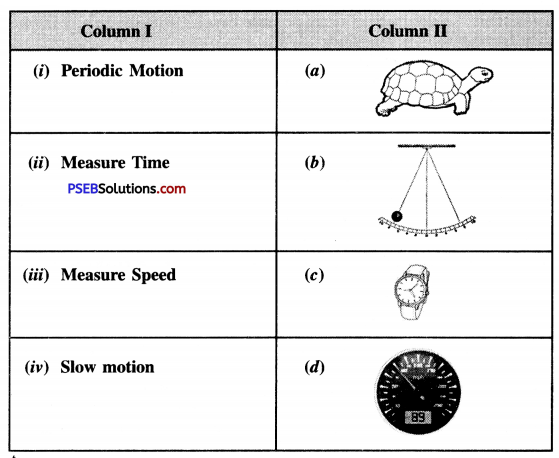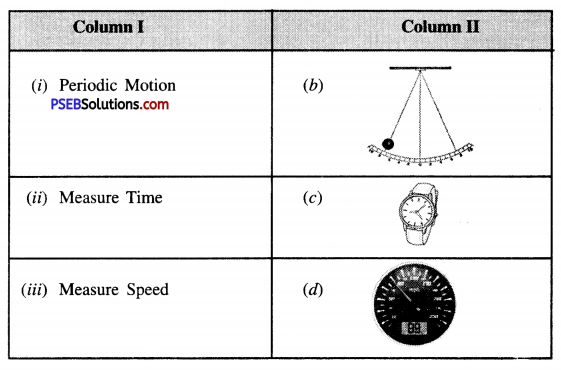Question (i)
Which of the following is a Distance-Time Graph for an object at rest ?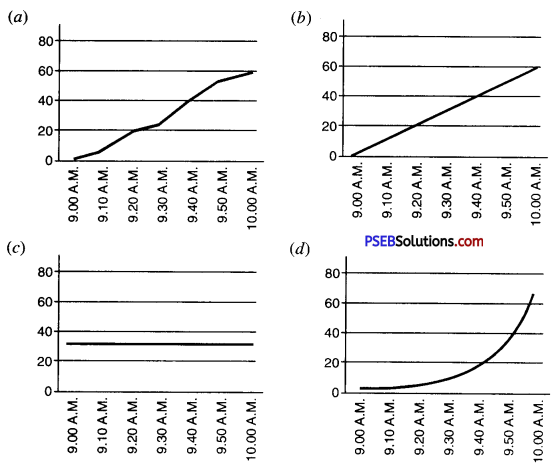(c) A straight line graph parallel to horizontal (x-axis) axisQuestion (ii)
Which of the following relation is a correct for finding speed of an object ?
(a) Speed = Distance × Time
(b) Speed = Distance/Time
(c) Speed = Time/Distance
(d) Speed = 1 /Distance × Time.
(b) Speed = Distance/Time

Question (iii)
Simple pendulum is an example of ……………….. motion.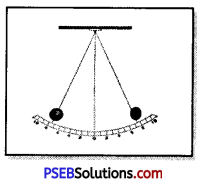(a) Rectilinear motion
(b) Oscillatory motion
(c) Periodic motion
(d) Rotational motion.
(c) Periodic motion

Question (iv)
A car moves with a speed of 40 km/h for 15 minutes and then with speed of 60 km/h for the next 15 minutes. The total distance covered by the car is:
(a) 100 km
(b) 25 km
(c) 15 Km
(d) 10 Km
(b) 25 km.

5. Very Short Answer Type Questions:

Question (i)
Define speed. Give its S.I. units ?
Speed. The distance travelled per unit time is called speed of the object.
Speed (v) = Total distance covered (s)/ Total time spent (t)
S.I. unit of speed is m/s.

Question (ii)
Which instruments were used for measuring time by the ancient people ?
In ancient times, people measured time by natural phenomena. Over time some devices were invented for measuring time. Some of them are given below:
(1) Solar Clock. A change in the position of the sun due to a change in the position of the shadow is used to measure time.
(2) Hour Glass. To measure the time it takes one hour for sand to flow from one bulb to another.
(3) Water Clock. Used to measure the flow of water from one vessel to another at a particular time.
(4) Pendulum. A simple pendulum takes equal time for each oscillation. So Pendulum was used to measure time.

Question (iii)
Name the instruments used for measuring : (a) the speed of a moving vehicle, (b) the distance moved by a vehicles.
(a) Instrument for measuring speed of a moving vehicle is Speedometer.
(b) Instrument for measuring distance covered by a vehicle is Odometer.

Question (iv)
What is Graph ? Give its types.
Graph. A graph is a pictorial representation of variation of one quantity with respect to the other quantity. When one quantity is changed, the value of the other quantity also changes by itself.

Types of Graph. There are several types of graphs, such as:

1. Linear graph,
2. Bar graph,
3. Pie chart.Question (i)
Differentiate between slow and fast motion. Give examples.
Slow motion. If an object takes too long to cover a short distance, its motion is called slow motion. Example : Motion of tortoise and snail.

Fast motion. If an object takes less time to cover the same distance, its motion is fast motion.
Example : Racing car and leopard is fast motion.

Question (ii)
Differentiate between uniform and non-uniform motion. Give examples.
Uniform motion.
If an object travels equal distances in equal interval of time regardless of the however small the interval may be their its motion is called uniform motion.
For example : A bus covers a distance of 10 km in the first 15 minutes and a distance of 10 km in the next 15 minutes, then again in the next 15 minutes it covers the same distance of 10 km. The bus is said to have uniform motion.

Non-uniform motion.
If an object does not cover equal distances in equal interval of time however small the time interval may be, then the motion of that object is called non-uniform motion.
Example : Suppose a car covers a distance of 25 km in 10 minutes on a straight road and travels 30 km in the next 10 minutes and 20 km in the next 10 minutes, then the car is said to have is non-uniform motion.

Question (iii)
Ajay goes to his school 600 meters away from his house. Find the speed in ms-1, with which he must walk to reach his school in 5 minutes.
Solution:
Distance from Ajay’s school from his house = 600 meters
Time taken to cover the distance from school to house = 5 minutes
We know 1 minute = 60 sec.
So, Time taken = 5 × 60 seconds
= 300 seconds
Total distance covered = 600
Now speed of Ajay =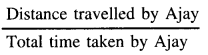= $$\frac{600 \text { metres }}{300 \text { second }}$$
= 2 m/s

Question (iv)
The distance between two stations is 216 km. In how many hours will a train reach the destination which is moving at a speed of 20 m/s ?
Distance between two stations = 216 km
Speed of train = 20 m/s. [∵ 1km/h = $$\frac {5}{18}$$ m/s]
= 20 x $$\frac {18}{5}$$ km/h
= 72 km/h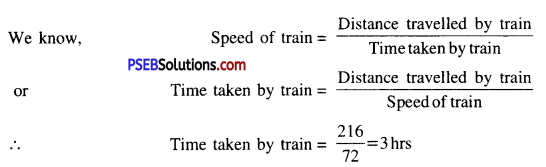Question (v)
Find the time period of a simple pendulum, which takes 20 s to complete 50 oscillations ?
Solution:
Number of oscillations of a simple pendulum = 50
Time taken to complete 50 oscillations = 20 seconds
We know, Frequency =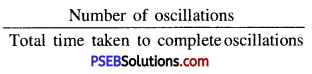= $$\frac {20}{50}$$
= $$\frac {2}{5}$$ = 0.4 sec-1

Question (i)
Describe the method to find the time period of a simple pendulum.
Make a simple pendulum by tying a metal sphere with one end of a thread about 1 meter long as shown in the diagram. If there is a fan running around, turn it off. Now let the bob of the pendulum come to rest in the middle position. Mark the middle position with chalk on the floor. Take a stop watch or mobile or wrist watch to measure the time period of the pendulum. Hold the bob and ensure that the thread is stretched and take it one extreme. Now release the sphere slowly. When the sphere is at its starting end, start the stop watch. Count the oscillations.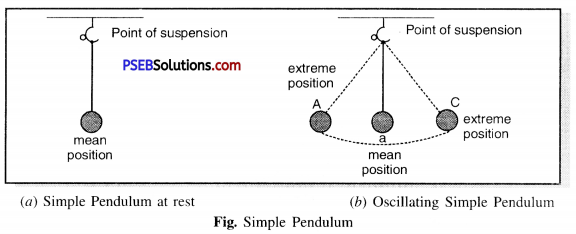Note the time taken by the pendulum to complete 20 oscillations. Record your observations in the table below. Time taken by the pendulum to complete one oscillation is called its time period. It is measured in second.

 Sr. No. Time taken for 20 oscillations Time period 1. 2. 3.

Repeat this action 3-4 times and note observations in the table above.
You will notice that the time period of pendulum will be almost the same each time.

Question (ii)
A car moves a distance of 60 km in 1st hour, 75 km in 2nd hour, 55 km in 3rd hour and 50 km in 4th hour. Plot a distance-time graph for the motion of the car.
(1) Find the speed of the car for the whole journey.
(2) Find the speed of the car between 1st and 3rd hour.
(1) Distance covered in the entire journey = 60 km + 75km + 55 km +50 km
= 240 km
And, Total time spent = 4 hours
The average speed of the car = The total distance covered/Total time spent
= 240 km/4 hr
= 60 km/hr

(2) Speed from the first hour to the third hour
Distance covered = 75 km + 55km
= 130 km
= 2 hours
Car speed between the first hour and the third hour =130 km/2hr = 65 km/hr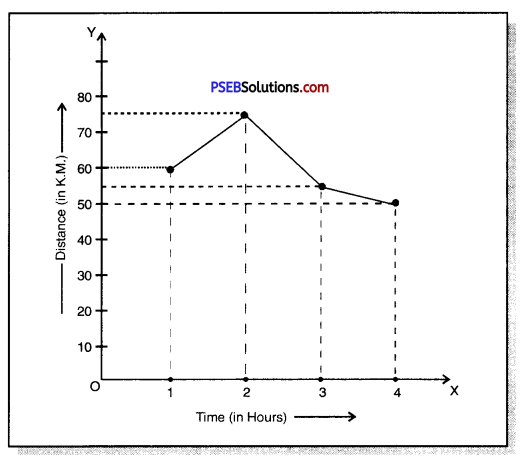Question (iii)
Figure shown below represents the distance-time graph for the motion of two vehicles A and B. Which one of them is moving faster ?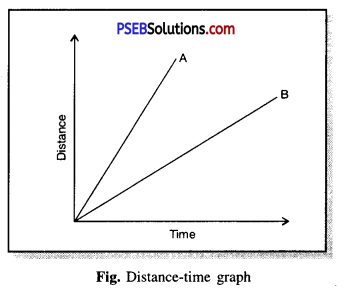Study of the distance-time graph of both the vehicles shows that the ratio of distance and time for vehicle A is higher than that of vehicle B, hence the speed of vehicle A is higher than that of vehicle B.

PSEB Solutions for Class 7 Science Motion and Time Important Questions and Answers

1. Fill in the Blanks:

(i) The distance covered by an object in unit time is called …………………
Speed

(ii) The time taken by a pendulum to make a swing is called ………………………..
Time period

(iii) An instrument used to measure the distance covered by vehicles is called ……………….
Odometer

(iv) …………………… is the instrument that measures the speed of moving vehicles.
Speedometer

(v) A graph drawn for the distance and time taken for a uniform motion is ………………………
Straight line parallel to time axis

2. Match the Column ‘I’ with Column ‘II’: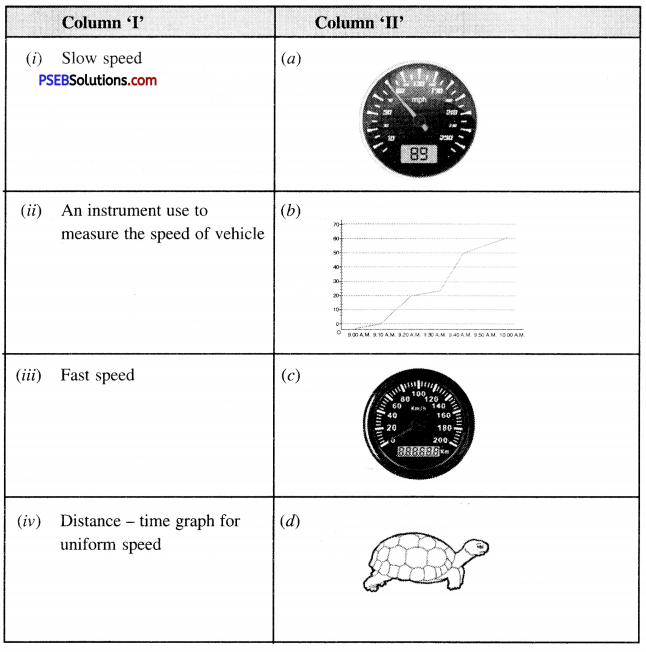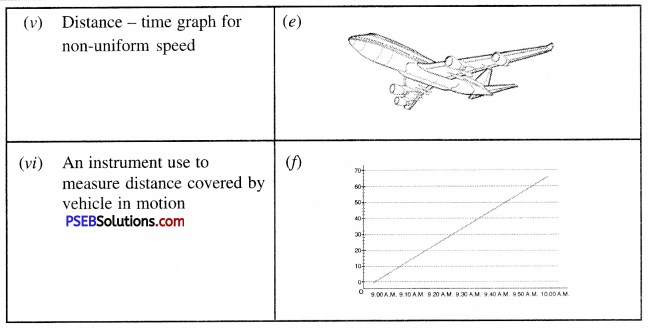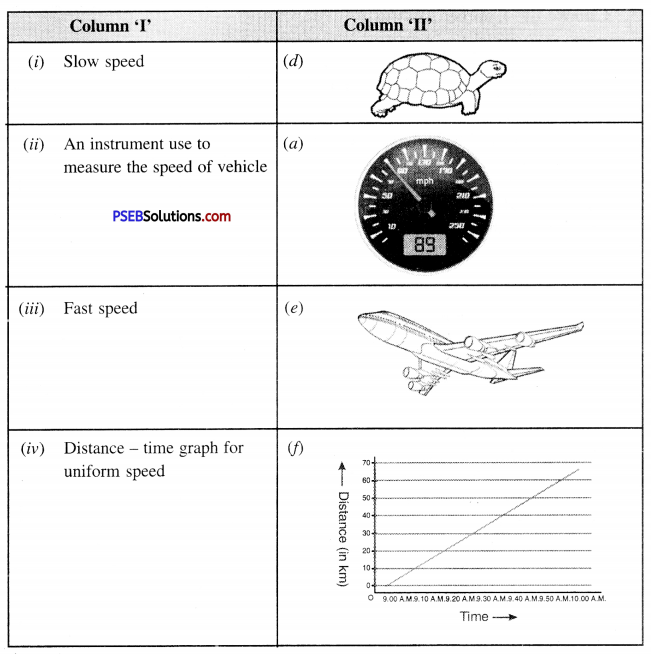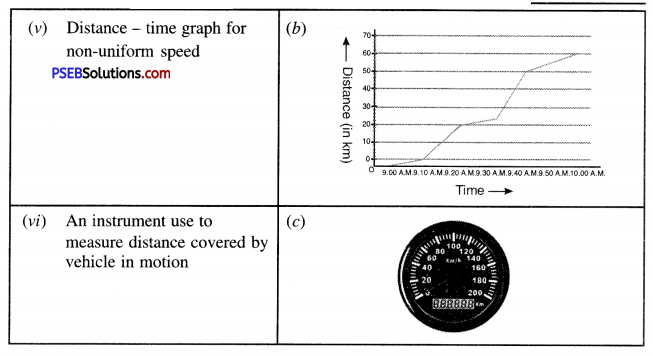Question (i)
The basic unit of time is:
(a) Minute
(b) Hour
(c) Second
(d) None of these.
(c) Second.

Question (ii)
The Speed of train is expressed in :
(a) m/h
(b) km/h
(c) cm/h
d) mm/h.
(b) km/h.

Question (iii)
The S.I. unit of speed is:
(a) m/s
(b) km/s
(c) cm/s
(d) mm/s.
(a) m/s.

Question (iv)
Which of the following relation is correct:
(a) Speed = Distance × Time
(b) Speed = $$\frac{\text { Distance }}{\text { Time }}$$
(c) Speed = $$\frac{\text { Time }}{\text { Distance }}$$
(d) None of these.
(b) Speed = $$\frac{\text { Distance }}{\text { Time }}$$

Question (v)
A motion which repeats itself after fixed interval of time is:
(a) Oscillatory motion
(b) Periodic motion.
(c) Time period
(d) None of these.
(b) Periodic motion.

Question (vi)
The device which measures the distance moved by the vehicle is called:
(a) Speedometer
(b) Odometer
(c) Pendulum
(d) None of these.
(b) Odometer.

Question (vii)
The time taken by a vibrating body to complete one oscillation is called:
(a) Time period
(b) Oscillation
(c) Uniform motion
(d) None of these.
(a) Time period.

Question (viii)
Which of the following Distance-Time Graph shows the object at rest position ?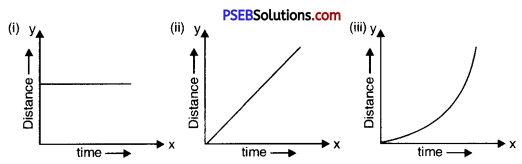(a) (i)
(b) (ii)
(c) (iii)
(d) None of the above.
(a) (i).Question (ix)
What is the basic unit of speed ?
(a) km/min.
(b) m/min.
(c) km/hr.
(d) m/s.
(d) m/s.

State True or False:

(i) The basic unit of time is second.
True

(ii) Every object moves with a constant speed.
False

(iii) Distances between two cities is measured in kilometres.
True

(iv) The time period of a given pendulum is not constant.
False

(v) The speed of a train is expressed in m/h.
False

Question 1.
The rate at which distance is covered corresponds to which quantity-speed or velocity ?
Speed.

Question 2.
The rate at which displacement is covered corresponds to which quantity, speed or velocity ?
Velocity.Question 3.
What is formula of average speed ?
Total distance covered
Average speed =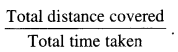Question 4.
Name two types of motions.
(i) Uniform motion
(ii) Non-uniform motion.

Question 5.
Working of clock depends on which type of motion ?
Periodic motion.

Question 6.
Name any two time measuring devices.
(1) Clock,
(2) watch.

Question 7.
What is metallic ball of a pendulum known as ?
Bob of the Pendulum.

Question 8.
What type of motion is shown by simple pendulum ?
Oscillatory motion / Periodic motion

Question 9.
What is basic unit of time ?
Second.

Question 10.
What are larger units of time ?
Minute, hourQuestion 11.
What is odometer ?
Odometer. The device which measures the distance moved by the vehicle, is called odometer.

Question 12.
What is function of speedometer ?
It records speed directly in km/h.

Question 1.
Which devices were used for measuring time before pendulum clocks ?
(i) Sundial.
(ii) Water clock,
(iii) Sand clock.

Question 2.
What important points are to be kept in mind while choosing the most suitable scale for drawing a graph ?
Points to be considered while choosing the scale for drawing a graph :
(0 The difference between the highest and the lowest values of each quantity.
(ii) The intermediate values of each quantity.
(iii) To utilize the maximum part of the graph paper.

Question 3.
What is a simple pendulum ?
Simple pendulum. It consists of a small metallic ball or a piece of stone suspended from a rigid support by a thread. The metallic ball is called the bob of the pendulum.

Question 4.
Classify the following as Linear, circular or Periodic motion :
(i) Motion of your hands while running.
(ii) Motion of a horse pulling a cart on a straight road.
(iii) Motion of a child in a merry go round.
(iv) Motion of a child over see-saw.
(v) Motion of the hammer of an electric bell.
(vi) Motion of a train on a straight bridge.
(i) Periodic motion
(ii) Linear motion
(iii) circular motion
(iv) Periodic motion
(v) Periodic
(vi) Linear Motion.

Question 5.
A simple pendulum takes 32 s to complete 20 oscillations. What is the time period of the pendulum ?
Solution:
Number of oscillations = 20
Time taken to complete 20 oscillations = 32 s
∴ Time period of pendulum =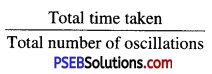(i.e. time taken to complete 1 oscillation) = $$\frac {32}{20}$$
= $$\frac {8}{5}$$
= 1.6 s

Question 6.
The distance between two stations is 200 km. A train takes 4 hours to cover this distance. Calculate the speed of the train.
Solution:
Distance covered by train between two stations = 200 km
Time taken t = 4 hr
∴ Speed of the train =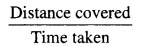= $$\frac{200 \mathrm{~km}}{4 \mathrm{hr}}$$
= 50 km/hr

Question 7.
Salma takes 15 minutes from her house to reach her school on a bicycle. If the bicycle has a speed of 2 m/s, calculate the distance between her house and her school.
Time taken = 15 min.
= 15 × 60
= 900 sec
Speed of bicycle = 2 m/s
Distance covered = Speed × Time
= 2 × 900 m
= 1800 m
= $$\frac{1800}{1000}$$
= 1.8 km.

Question 1.
Define
(i) Periodic motion
(ii) Oscillatory motion
(iii) Oscillation
(iv) Time period.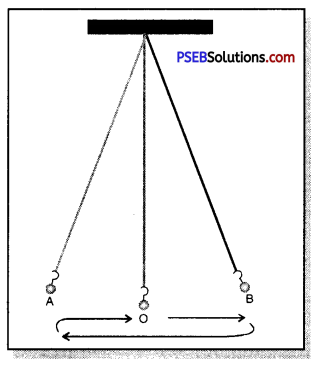(i) Periodic motion. A motion which repeats itself after fixed interval of time is periodic motion.
(ii) Oscillatory motion. To and fro motion of an object about a fixed mean position is oscillatory or vibratory motion.
(iii) Oscillation. The motion of the pendulum from one extreme position to another extreme position and back to the first extreme position is one complete oscillation.
(iv) Time period. The time taken by a vibrating particle to complete one oscillation is called time period of the pendulum.Motion and Time PSEB 7th Class Science Notes

• If an object does not change its position with respect to the objects around it and with time, then that object is called at rest.
• If an object changes its position according to the objects around it and with time, then it is in motion.
• The motion in a straight line of an object is called straight line motion.
• The motion of an object on a circular path is called circular motion.
• If an object moves around its center position, the motion of that object is called Periodic motion.
• If an object takes very little time to cover a short distance then the speed of the object is fast and if the object takes more time to cover the same distance then its speed is called slow.
• The distance covered in 1 unit time is called the Speed. Its S.I. unit is meter per sec (m/s).
• The Speed can be calculated using the following formula : ccc
• The motion of an object moving at a speed along a straight line is called a Uniform Motion, when the motion of an object moving at different speeds on a straight line is called Non uniform Motion.
• The motion of hour hand of the clock, the motion of the earth around the sun, and the motion of a simple pendulum all are examples of uniform motion.
• Nowadays we measure time with wall clocks, table clocks, wrist watches or mobile phones. The SI unit of time is seconds.
• Heavy mass (metal sphere) tied to a thread and hung in a fixed place or stand is called a simple pendulum.
• movement of a simple pendulum back and forth a point is called a recurring or oscillating motion.
• The time taken to complete a one oscillation is called time period.
• The number of oscillations completed in a second is called frequency. The S.I. unit of frequency is hertz.
• A device that measures the speed of vehicles is called a Speedometer.
• The speedometer measures the speed of vehicles in kilometres / hour.
• An instrument used to measure the distance covered by vehicles is called an Odometer.
• Graph illustrates the comparison of one quantity with another. There are generally three types of graphs prevalent : (1) Linear graph, (2) Bar graph, (3) Pie chart
• The distance time graph is a linear graph. It represents the graph between the distance and time covered by the object. The amount that is independent is represented on X- axis (horizontal axis) and the other amount
• which is dependent is on the Y-axis (vertical axis).
• If an object is at rest, its distance-time graph is a straight line parallel to the X-axis.
• Graph: If two quantities depend on each other then their representation is called a graph.
• Speed: The distance covered by the object in the unit time interval is called the speed.
• Uniform motion: When an object travels equal distances in equal time intervals, the motion is uniform.
• Non uniform motion: The motion of an object when it does not cover the same distance in equal time intervals is called non uniform-motion.
• Simple pendulum: A small piece of metal or stone hanging with the help of thread from a fixed point is called simple pendulum.
• Oscillation: When a freely hanging object moves from its center position to one extreme side and then to the other extreme side and finally reaches its previous position i.e. middle position, the object is said to completes a oscillation.
• Time period: The time taken by a simple pendulum to complete a oscillation is called a time period.
• Uniform speed: When an object travels equal distances in equal time intervals, no matter how small the time interval, the speed of the object is called uniform speed.
• Non-uniform speed: When an object does not cover equal distances in equal time intervals, no matter how short the time interv al, the speed of that object is called non-uniform speed.

Punjab State Board PSEB 7th Class Science Book Solutions Chapter 13 Motion and Time Textbook Exercise Questions and Answers.